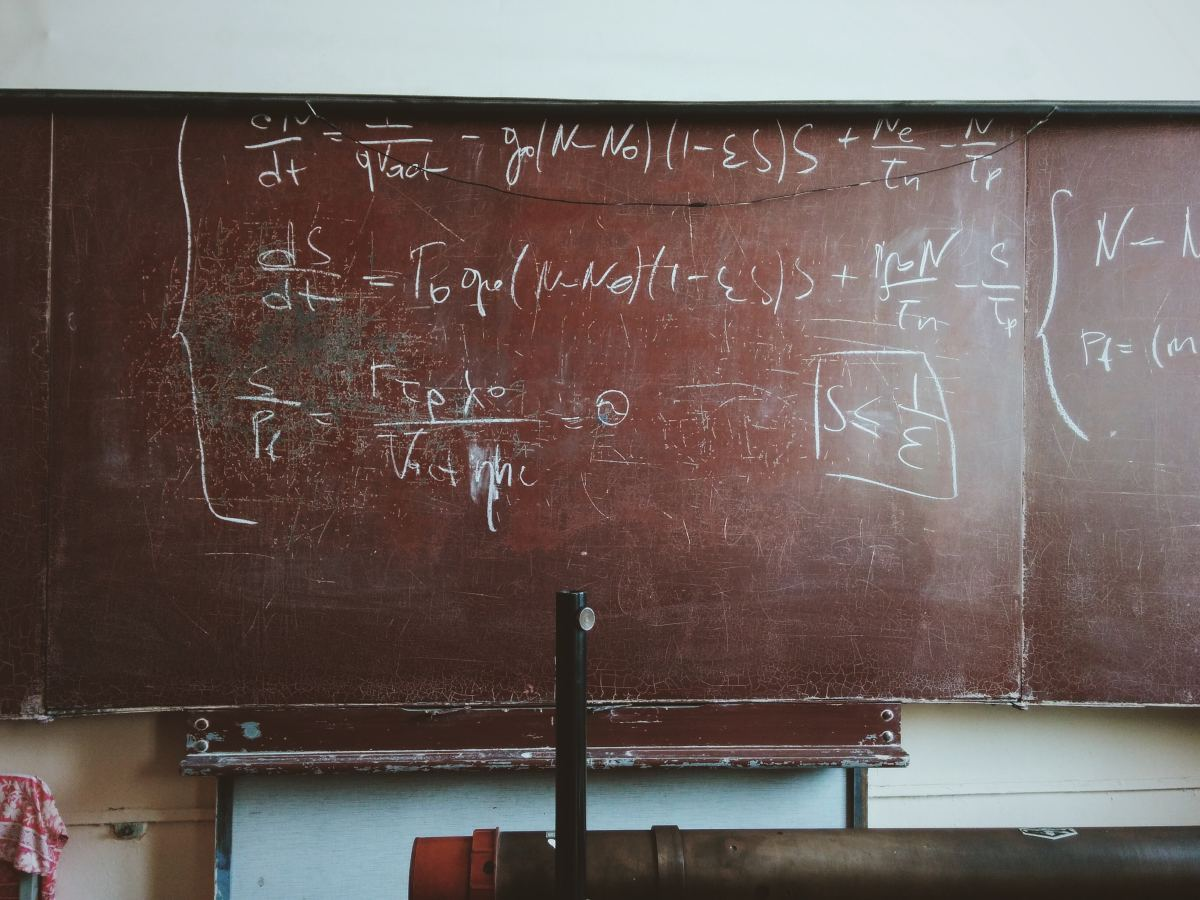# Solving Word Problems Involving Chebyshev's Theorem

A graduate of Master in Education with Mathematics as major from the De La Salle University- Manila, Philippines. A PRC licensed teacher.Learn how to solve word problems involving Chebyshev's theorem.Roman Mager, via Unsplash

## Four Problems Solved Using Chebyshev's Theorem

Chebyshev’s theorem states that the proportion or percentage of any data set that lies within k standard deviation of the mean where k is any positive integer greater than 1 is at least 1 – 1/k^2.

Below are four sample problems showing how to use Chebyshev's theorem to solve word problems.

## Sample Problem One

The mean score of an Insurance Commission Licensure Examination is 75, with a standard deviation of 5. What percentage of the data set lies between 50 and 100?

First, find the value of k.

Mean – (k) (sd) = lower limit

75 – 5K - 50

75 – 50 = 5k

25 = 5k

K = 5

To get the percentage, use 1 – 1/k^2.

1 - 1/ 25 = 24/25 = 96%

Solution: 96% of the data set lies between 50 and 100.

## Sample Problem Two

The mean age of a flight attendant of PAL is 40 years old, with a standard deviation of 8. What percent of the data set lies between 20 and 60?

First, find the value of k.

Scroll to Continue

40 – 20 = 8k

20 = 8k

k = 2.5

Find the percentage.

1 – 1/(2.5)^2 = 84%

Solution: 84% of the data set lies between the ages 20 and 60.

## Sample Problem Three

The mean age of salesladies in an ABC department store is 30, with a standard deviation of 6. Between which two age limits must 75% of the data set lie?

First, find the value of k.

1 - 1/k^2 = ¾

1 - ¾ = 1/k^2

¼ = 1/k^2

k^2 = 4

k = 2

Lower age limit:

30 - (k ) (sd) = 30 - (6)(2) = 30 -12 = 18

Upper age limit:

30 + ( k) (sd) = 30 + (6)(2) = 30 + 12 = 42

Solution: The mean age of 30 with a standard deviation of 6 must lie between 18 and 42 to represent 75% of the data set.

## Sample Problem Four

The mean score on an accounting test is 80, with a standard deviation of 10. Between which two scores must this mean lie to represent 8/9 of the data set?

Find first the value of k.

1 - 1 /k^2 = 8/9

1 - 8/9 = 1/k^2

1/9 = 1/k^2

k^2 = 9

k = 3

Lower limit:

80 – (10)(3) = 80 – 30 = 50

Upper limit:

80 + 30 = 110

Solution: The mean score of 60 with a standard deviation of 10 must lie between 50 and 110 to represent 88.89% of the data set.

RABIA AMINA on April 28, 2020:

i like it too much

Cristina Santander (author) from Manila on June 01, 2019:

Thanks to all those who appreciated this hub. Stay blessed.

Marie on May 31, 2019:

Thank you

Cristina Santander (author) from Manila on March 12, 2019:

thanks.

Shameem Tahseen on March 12, 2019:

Nice and simple examples

Cristina Santander (author) from Manila on March 09, 2019:

Thanks for appreciating this hub.

DAVID KASIRYE-MSc. Development Economics, UGANDA MARTYRS UNIVERSITY on March 07, 2019:

Fantastic lesson

Cristina Santander (author) from Manila on January 29, 2019:

Thanks for appreciating this hub.

SHIVANI TIWARI on January 28, 2019:

Thankyou for uploading it. Very useful and clear all my doubts regarding this theorem

Cristina Santander (author) from Manila on July 07, 2018:

Thanks for appreciating this hub. Good day !

thanks for appreciating this solution on June 04, 2018:

Thanks for appreciating this solution

seid hansar on June 04, 2018:

i like it

Cristina Santander on May 11, 2018:

Thanks for dropping by. Stay blessed.

saleem on May 11, 2018:

this is very usefull for me

thanks author of this site

Steve Sebastian (KNUST) on April 02, 2018:

Wow, to be frank this by far the best site for chebyshev's theory explanation. Examples are on point and clear. I really love this site

Sijo on May 12, 2016:

Thank you for the questions Ma'am

JP Carlos from Quezon CIty, Phlippines on February 09, 2012:

I like math though I'm not good at it. I use stat especially when I do my Training Needs analysis. I need to back up my training proposals with hard facts. Statistics often gets the training approved.

Cristina Santander (author) from Manila on February 09, 2012:

Hi jpcmc I am glad to hear from you again. Great to see you on this hub. Thank you for dropping by and appreciating this hub. Your visit and comments are much appreciated. Sometimes we have to do the old fashioned manual computation to appreciate Statistics better. I find Statistics a very practical Mathematics. Statistics has sought wide range of applications in business and even in our day to day activities. Blessings to you always and to your family. Best regards.

JP Carlos from Quezon CIty, Phlippines on February 09, 2012:

This brings back painful memories. But it's nice to review numbers once again. I just don't know why I took an extra elective in math class in 3rd year high school. I'm a certified nerd!

But seriously, I use SPSS for any staistical computation I need for work. Of course doing it the old fashioned manual computation is important.

Thanks for the clear discussion. I need to bookmark this hub.